International Journal of Science and Qualitative Analysis
Volume 1, Issue 3, September 2015, Pages: 64-68

I. Hamidipoor1, *, N. Golsanamlou2, I. Zare3, P. Moradi4

1Department of Mechanical Engineering, Miami University, Ohio, USA

3Department of Cell and Molecular Biology, Semnan University, Semnan, Iran

4Department of Mechanical Engineering, Jahrom University, Jahrom, Iran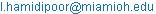(I. Hamidipoor)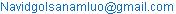(N. Golsanamlou)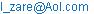(I. Zare)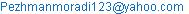(P. Moradi)

I. Hamidipoor, N. Golsanamlou, I. Zare, P. Moradi. The Impact of Blade and Material Damping in Turbine Blades. International Journal of Science and Qualitative Analysis. Vol. 1, No. 3, 2015, pp. 64-68. doi: 10.11648/j.ijsqa.20150103.14

Abstract: A method of determining equivalent viscous damping ratio for different rotational speeds and modes as a function of displacement or strain at a reference point in a blade is presented. This method can be adopted using a suitable finite element code, e.g., ANSYS or ABAQUS. The friction damping concept is widely used to reduce resonance stresses in turbomachines. A friction damper has been designed for high pressure turbine stage of a turbojet engine. The objective of this work is to find out effectiveness of the damper while minimizing resonant stresses for sixth and ninth engine order excitation of first flexure mode. The capability to assess damping in the blade from finite element codes avoids costly tests at the initial stages of design.

Keywords: Friction Damping, Turbine, Resonant Stress, Blade, Vibration, Friction Coefficient

Contents

1. Introduction

Blade fatigue failures are very common in turbomachinery. When the frequency of any of these harmonics coincide with any one of the natural frequencies, resonance occurs. The high stresses at resonance lead to fatigue problems. The only way by which the resonant stresses can be controlled is by dissipation of the vibratory energy. Thus damping becomes an important aspect of the blade design.

For a turbine blade the means by which this vibratory energy could be dissipated is by material damping, Coulomb damping at the interfaces, gas dynamic damping and possibly impactive damping. All these damping mechanisms are complex and non-linear in nature. Generally the aerodynamic damping is very low, and when there is no impact damping, the material and Coulomb damping between interfacial slipping surfaces are the main sources of dissipation of energy. A designer usually depends on past experience and test results to estimate the available damping in limiting the resonant stresses. Attempts have been made to quantify the material damping using Lazan’s damping law or by contact elements using 2D models 

The friction damping concept is widely applied in turbomachinery applications, especially at hot end parts, to reduce resonance stresses. A typical application of this in gas turbines. They are popularly called as "friction damper," "cottage-roof damper" or "under platform damper." This damper is loaded by centrifugal force against the underside of the platforms of two adjacent blades. The main design criterion for such devices is to determine the optimum damper configuration or the damper mass or both in order to reduce the dynamic stresses to maximum possible extent.

For example, if the damper mass is too small for a given configuration, the friction force will not be large enough to dissipate sufficient energy. On the other hand, if the damper mass is too large, it will get into "stick" condition, thereby limiting the relative motion across the interface and hence the amount of energy dissipation. In both cases, the friction damper will be inefficient, and between these two extremes there exists an optima.

However, an application of these procedures to determine the equivalent viscous damping values in turbine blades is not successfully implemented.

Based on experimental work, Rao et al.  quantified blade damping as a function of strain amplitude in each mode of vibration for different speeds. This non-linear model of damping has been successfully used in evaluating the resonant stresses . In this research a novel method is clarified in order to estimate material damping, friction damping as well as combined material and friction damping using a finite element code, ANSYS.

2. Material Damping

Rowett  investigated the torsional damping properties of certain grades of steel shafting and suggested an early form of stress damping law,(1)

Where EH is energy loss per unit volume, J is a constant of proportionality, s is the local stress and n is a damping exponent.

The total energy dissipated by material hysteresis is obtained by integrating the above stress relationship throughout the structure. E. P. Petrov and D. J. Ewins  conducted comprehensive studies into the general nature of material damping and presented damping results data for almost 2000 materials and test conditions. Their result clearly clarified that the logarithmic decrement values increase with dynamic stress, i.e., with vibration amplitude, where material damping is the dominant mechanism.

To determine the resonant stress of a turbine blade passing through a critical speed, the most practical approach is to use a viable commercial code such as ANSYS. Damping plays an important role in determining this stress value accurately and experimentally, Rao et al.  demonstrated a method of quantifying the equivalent viscous damping in each mode at different rotational speeds. Rao et al.  used such a model to obtain the resonant stresses. A procedure to determine the equivalent viscous damping by computational means is demonstrated below.

Non-Linear Viscous Damping Due to Material Hysteresis

A simple rectangular blade (Sandvik O&T steel) 5 cmX1 cm with a T root is considered for the study. The blade length is 30 cm. The contact surfaces on either side are 5 cmX1 cm. The disk is taken to be 14 cm long and 25 cm wide with 1 cm thickness for the purpose of modelling and the contact surfaces are 4 cm from the top of the disk. The blade root is taken as 15 cmX5 cm. The blade and its root are modelled using 606 SOLID 45 elements . The interfacial surfaces between the blade root and disk are modelled as 20 CONTACT 173 and 20 TARGET 170 contact elements. A friction coefficient 0.4 is assumed between the contact elements. The material density is 7800 kg/m3. The elastic modulus is taken as 210 GPa. The material damping property is taken from Ref.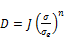(2)

Where D=specific damping energy kNm/m3/cycle, J=16.0, n=2.3, and σe=fatigue strength=630 Mpa. Using Lazan’s law above and the material properties, the coefficient J and exponent n; the specific damping energy, total damping energy and the total strain energy are calculated by integrating over the entire volume. The strain amplitude at a reference point near the root of the blade is monitored throughout the analysis. The loss factor, ratio of total damping energy and total strain energy is obtained. It is then converted to equivalent viscous damping for the strain amplitude considered at the reference point. The mode shape is then modified to have different values of strain amplitude at the reference point and the specific damping energy, total damping energy and the total strain energy are correspondingly updated. Thus, the equivalent viscous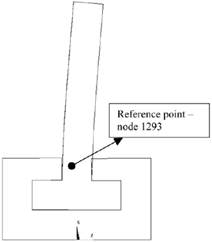Fig. 1. The chord wise mode 372.06 Hz of the blade at 200 rpm.

Damping is determined as a function of strain amplitude at the reference point for the given mode and speed. The same procedure can be applied to obtain the equivalent viscous damping values for other modes at the same speed. Here, only one mode is considered to illustrate the procedure.

The steps in calculation are illustrated below for 200 rpm. With the help of ANSYS, the natural frequencies and orthonormal mode shapes are first determined. For determining the material damping characteristics the contact elements are closed.

The first two natural frequencies are 82.6 and 383.67 Hz. For a similar case, considered the chord wise mode to determine friction damping [1,9]. Here the second mode corresponding to the chord wise direction is considered to illustrate the procedure. This mode shape is shown in Fig. 1. To estimate the damping in each mode the following procedure is adopted.

Total damping energy:

The total damping energy D0 (Nm) is given by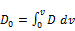(3)

Where v is the volume.

Loss factor ɲ: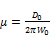(4)

Where W0 is the total strain energy (Nm).

Equivalent viscous damping :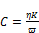(5)

Where C is the equivalent viscous damping (N s/m), ω is the natural frequency (rad/s), K is the modal stiffness (N/m).

To obtain the equivalent viscous damping at other strain amplitudes a program is written in Microsoft Excel taking advantage of the proportionality of stress and strain energy with the strain amplitude. For increased strain amplitudes, the orthonormal reference strain amplitudes, stress and strain energy are multiplied by a factor F to obtain the equivalent viscous damping Ce at various strain amplitudes. The procedure is best illustrated by the following numerical calculations: natural frequency in the first mode, ωn = 2411.93 rad/s; orthonormal strain amplitude at the reference point—node 1293, see Fig. 1, ε = 0.269826; total damping energy, D0 = 214235.4 Nm; strain energy, W0 = 2908699 Nm (ANSYS output); loss factor, ɳ = D0/(2πW0) = 0.011722; equivalent viscous damping (Ns/m), Ce = ɳK/ ωn = 28.2733; damping ratio,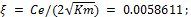where m = 1 and K = (ωn)2the strain amplitude is multiplied by a factor F = 0.1 then the corresponding strain amplitude is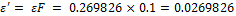; the total damping energy is; the strain energy is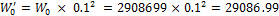; the loss factor is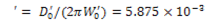; the equivalent viscous damping is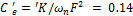; whereand the damping ratio is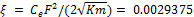: The damping characteristic thus obtained is shown in Fig. 2. In a similar manner the damping characteristics can be obtained at other speeds. Since the chordwise mode is not significantly affected by centrifugal load, the damping characteristic remains almost the same as given in Fig. 2.Fig. 2. Material damping given as equivalent viscous damping ratio as a function of reference strain amplitude for the chordwise mode.

3. Friction Damping

To determine the equivalent viscous damping from friction at the interfaces between the disk and root, the omega load corresponding to the desired speed is given, e.g., 200 rpm. An impulse, say 60 N, is then applied at the blade tip in the required (chord wise) direction to excite the chord wise mode. The response thus obtained is used to determine the friction damping as a function of reference point displacement. A typical decay curve obtained is shown in Fig. 3. The damping due to friction is calculated from this response decay curve .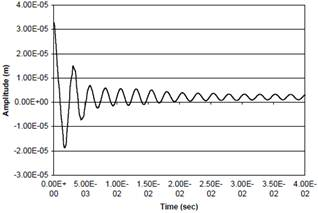Fig. 3. Free vibration decay due to friction at 200 rpm.

Initially, when the displacements are large, the damping is high and the decay is rapid .

Subsequently the decay envelope is a straight line following Coulomb’s law . Using this decay curve, the equivalent viscous damping in the system as a function of the strain amplitude at the reference point is generated. Fig. 4 gives the equivalent viscous damping as a function of the reference displacement at 200 rpm. At higher speeds, the interfacial slipping disappears as the contact elements get closed. The free vibration decay curve at 800 rpm is given in Fig. 5. It can be inferred that there is practically no damping in the system due to friction.Fig. 4. Equivalent viscous damping as a function of amplitude at 200 rpm due to interfacial friction.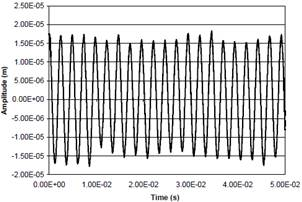Fig. 5. Free vibration decay at 800 rpm due to interfacial friction.

4. Combined Material and Friction Damping

To determine the combined effect of material and interfacial friction, the average equivalent viscous damping from the material damping run is included in the response calculation due to friction as discussed above. The resulting net equivalent viscous damping values are then obtained to define the non-linear damping behavior of the bladed-disk system.

The results from the above investigation are presented in graphical form in Fig. 6.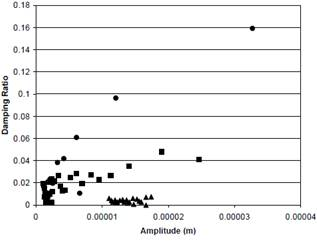Fig. 6. Combined friction and material damping results for 200, 500 and 800 rpm: ●200 rpm; ■ 500 rpm; ▲ 800 rpm.

As can be seen from Fig. 6, the influence of friction gradually disappears as the speed increases and only material damping is available at high speeds. The effect of material damping over friction at a low speed, 200 rpm is presented in Fig. 7.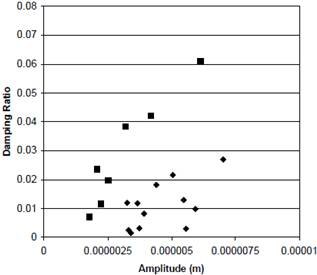Fig. 7. The effect of material damping over friction damping at 200 rpm: ♦ friction damping; ■ friction and material damping.

5. Conclusion and Future Works

From the title of this paper, it can be inferred that the minimization of resonant stresses in turbine blades is a major concern in turbine engine. A simple procedure is outlined for optimization of damper for turbine blade so that the maximum stress experienced by any blade should be below some designated maximum value.

A method of determining equivalent viscous damping ratio for different rotational speeds and modes as a function of displacement or strain at a reference point in a blade is presented. This method can be adopted using a suitable finite element code, e.g., ANSYS or ABAQUS (ANSYS was used in this paper). The capability to assess damping in the blade from finite element codes avoids costly tests at the initial stages of design. It is shown that the effect of friction disappears with increasing speed and that the viscous damping ratio increases with strain in the blade.

The predicted displacements, stress distribution natural frequencies determined for the 1st flexure mode of the blade is carefully clarified. The damper performance which is used to arrive at optimal damper design. This can now be extended to the engine condition, that is, temperatures, and gas loads.

The non-linear model developed here can be easily applied in order to govern the resonant response very accurately and consequently improve the current design capabilities in blade life estimation.

References

1. J.S. Rao, Turbomachine Blade Vibration, Wiley, New York, 1991.
2. J.S. Rao, N.S. Vyas, K. Gupta, Blade damping measurement in a spin rig with nozzle passing excitation simulated by electromagnets, Shock & Vibration Bulletin 56 (pt 2) (1986) 109.
3. J.S. Rao, N.S. Vyas, Determination of blade stresses under constant speed and transient conditions with nonlinear damping, Journal of Engineering for Gas Turbines and Power, Transactions American Society of Mechanical Engineers 118 (2) (1996) 424.
4. F.E. Rowett, Elastic hysteresis in steel, Proceedings of the Royal Society 89 (1914).
5. E. P. Petrov and D. J. Ewins, "Advanced modeling of underplatform friction dampers for analysis of bladed disk vibration," Journal of Turbomachinery, vol. 129, no. 1, pp. 143–150, 2007.
6. J.S. Rao, A.K. Singh, C.V. Ramakrishnan, K. Gupta, The effect of nonlinear damping on the resonant stresses in turbomachine LP rotor blades, I Mech. E. Vibrations in Rotating Machinery, Conference Transactions, 12–14 September 2000, Nottingham, UK, p. 45.
7. ANSYS workbench 14.0, ANSYS Inc., Canonsburg, PA.
8. C.M. Harris, C.E. Crede, Shock and Vibration Handbook, McGraw-Hill, New York, 1976.
9. Gui N, Fan JR, Cen KF, et al. A direct numerical simulation study of coherent oscillation effects of swirling flows. Fuel 2010; 89: 3926–3933.
10. C. W. Schwingshackl et al., "Measured and estimated friction interface parameters in a nonlinear dynamic analysis," Mechanical Systems and Signal Processing, vol. 28, pp. 574–584, 2012.
11. J. Szwedowicz, C. Gibert, T. P. Sommer, and R. Kellerer, "Numerical and experimental damping assessment of a thin walled friction damper in the rotating setup with high pressure turbine blades," Journal of Engineering for Gas Turbines and Power, vol. 130, no. 1, Article ID 012502, 2008.
12. R. K. Giridhar, P. V. Ramaiah, G. Krishnaiah, and S. G. Barad1, Gas Turbine Blade Damper Optimization Methodology, Advances in Acoustics and Vibration Volume 2012, Article ID 316761, 13 pages doi:10.1155/2012/316761.

 Contents 1. 2. 3. 4. 5.
Article ToolsAbstractPDF(296K)